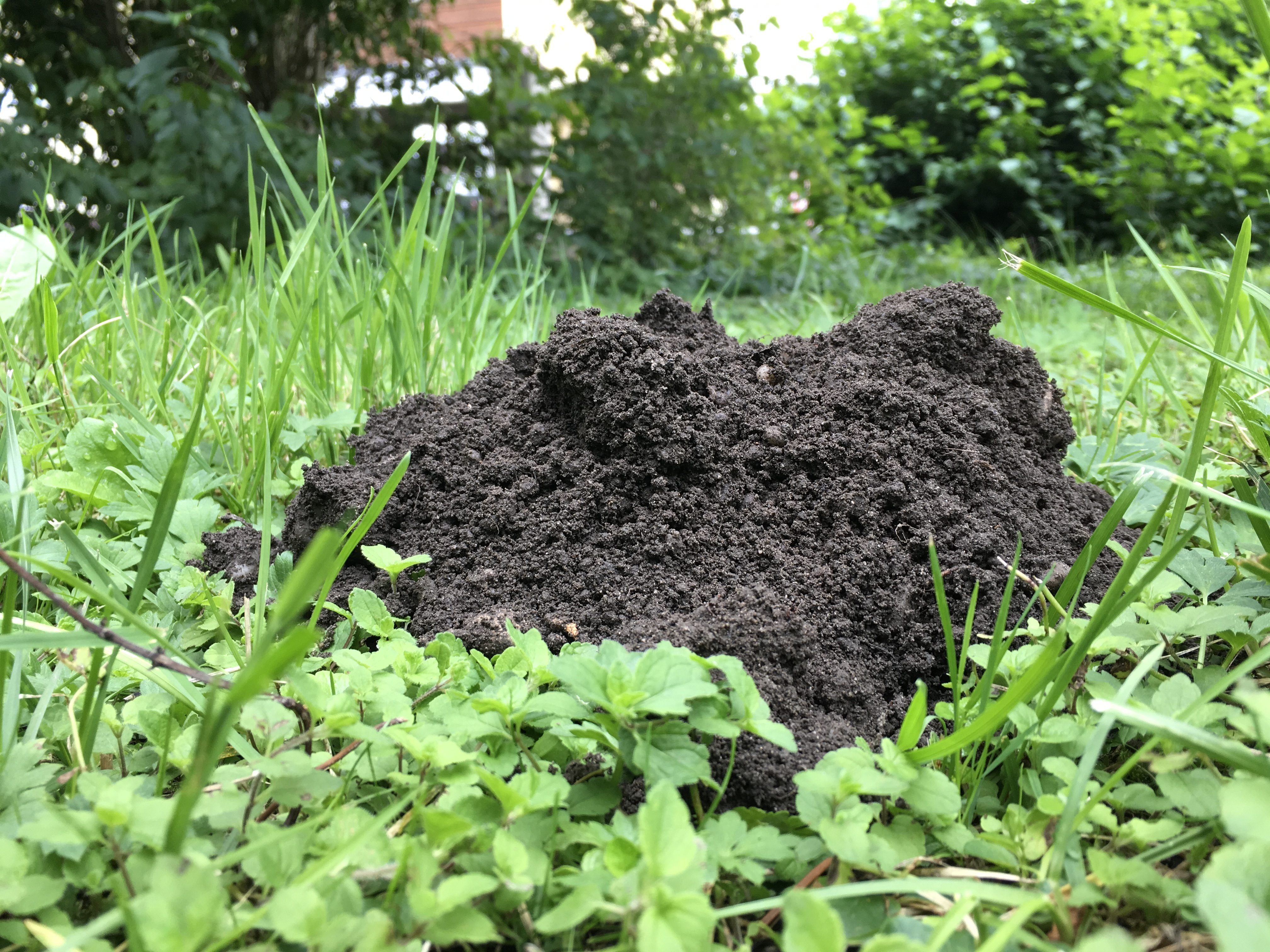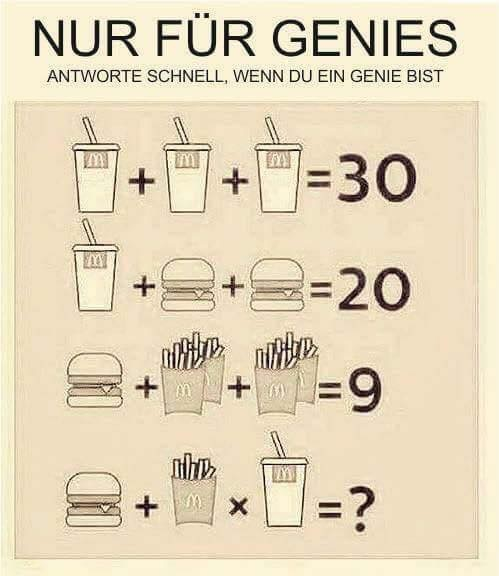# What makes an equation beautiful

## 13. Solve equations

You can transform linear equations until the solution is visible.

### Words 1

the same, the equation, solve, the solution, the page, different, see, look, change, read, read off, write, the bracket, swing, the amount, the solution amount, expect, hide, play, hide and seek, look, beautiful , look, look, learn, shape, transform (= change), the equivalence (equivalence)Hide and seek with variables: if a variable is hidden in an equation, then we can look for it What does she look like? What can we expect here? This is particularly nice to learn with linear equations. We'll look at that in this chapter.

### 1. Solve an equation

An equation has two sides that are the same. Even if they look different.You may be familiar with this task from the Internet.
What is the solution? (Source: unknown)

### solution

Drink: 10, burger: 5, fries: 1, burger + fries * drink = 15### example

We'll modify the equation until it looks like we can easily read the solution. The aim of these changes is that x is alone on the left. We always do the opposite of what bothers us. On both sides. Such changes are called "equivalent transformations". The solution found is correct for each of the resulting equations.

We write the solution between curly brackets and call this the solution set L.

### Words 2

the equation, the variable, simple, complicated, even, the value, accept, agree, linear, quadratic, the degree, the basis, actually, employ, test, set, insert, make

An equation has one or more variables. It can be simple and complicated. It can have zero, one, or even multiple solutions. A solution is a number. If the variable takes the value of this number, then the equation is correct.

We only want to deal with solving linear equations in these fundamentals. How do we actually know whether our solution is correct? This is shown by the sample.

### 3. The rehearsal

For the sample, we plug 7 into the equation.

7 really is a solution. We did the test.

### Exercises

Try the exercises above.

### 4. Outlook

We'll keep looking at linear equations. In the next chapter we will see what they have to do with everyday life. In further math courses we will see that we can also “draw” these equations. For a start, however, what we have done here in this chapter is sufficient. It's great that you're here.

Corrections: Maria Fatoba

Found a mistake? Please send an email to [email protected] Many Thanks!